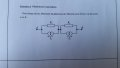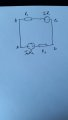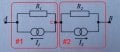# Merging 2 norton sources that are in series with one another

Joined Dec 31, 2014
25
Hello,

I've just had my first classes of electronics and I have some homework that you can find in the attachments as well as my solution to it. The exercice states: "For each circuit, determine the sources of Thevenin and Norton, seen from A & B.". I am quite confused as to how this all works so could you give me some detailed explenation on why what I did is wrong if it is.

Vlad#### WBahn

Joined Mar 31, 2012
29,480
Your two Norton sources are not connected between A and B. One is connected between A and C (the middle node) and the other is connected between C and B. Your transformed sources need to honor those connections.

#### DGElder

Joined Apr 3, 2016
351
The question is somewhat ambiguous so I am not sure what is expected of you. I would simplify the circuit by
looking into terminals A and B and create the single Norton and then the single Thevenin equivalent generator.

#### crutschow

Joined Mar 14, 2008
33,323
You calculate the voltage between A and B for the given current source values for the circuit as originally shown using loop equations or other method of your choice.
Then you calculate the resistance between A and B.
From that you can generate the equivalent Thevenin and Norton equivalents.

#### DGElder

Joined Apr 3, 2016
351
You have no expression now for the Vth voltages

Joined Dec 31, 2014
25
You have no expression for the Vth voltages
Uac = I1R1 and Ubc = I2R2?

#### crutschow

Joined Mar 14, 2008
33,323
That's not correct. You can't remove the middle connection.
It cannot be reconfigured into a simpler form so stop trying.

You need to calculate the voltage and resistance for the original circuit, as I stated.

Only after that can you reconfigure the circuit into its Thevenin and Norton equivalents.

#### DGElder

Joined Apr 3, 2016
351
Uac = I1R1 and Ubc = I2R2?
Correct. Now I would simplify into one Thevenin Equivalent. And then I would change that to a Norton equivalent.

Or you could have just gone straight to a single equivalent without breaking in a C node, as I and Crutschow suggested.

Then you have covered all the approaches, required or not.

Last edited:

Joined Dec 31, 2014
25
Correct. Now I would simplify into one Thevenin Equivalent. And then I would change that to a Norton equivalent.

Or you could have just gone straight to a single equivalent without breaking in a C node, as I and Crutschow suggested.

Then you have covered all the approaches, required or not.
Ok, forgive me for being so slow but I am completely new to this and it's hard to wrap my head around.
I have Uac and Ubc which is great and I assume that Uab would be Uac + or -Ubc because I know that the sign of the voltage depends on whether it goes against (+) or in the same direction (-) as the current. But what is the direction of this current? It's not marked on the paper.

#### DGElder

Joined Apr 3, 2016
351
Ok, forgive me for being so slow but I am completely new to this and it's hard to wrap my head around.
I have Uac and Ubc which is great and I assume that Uab would be Uac + or -Ubc because I know that the sign of the voltage depends on whether it goes against (+) or in the same direction (-) as the current. But what is the direction of this current? It's not marked on the paper.
Current directions are defined in the question. From that you got the polarity of Uac and Ubc - per the Passive Sign Convention. From your sketch you have decided that the first subscript letter indicates the positive terminal and the second subscript the negative terminal. Being consistent in the output ab: Uab = Uac-Ubc = Vth. Makes sense?

Last edited:

Joined Dec 31, 2014
25
What I got is:

Uab = Uac - Ubc = Vth
Rth = R1 + R2
In = (I1R1 - I2R2) / (R1 + R2)

Is this what you and crutschow were trying to tell me? I hope I got it right this time.

#### Attachments

• 2.8 MB Views: 4

#### crutschow

Joined Mar 14, 2008
33,323
......................
But what is the direction of this current? It's not marked on the paper.
The arrow next to the current source shows the current direction.
The loop current direction is determined by that.

•Joined Dec 31, 2014
25
Of course the arrow shows the current for the loop, but what about the whole circuit? Like what enter A and goes out through B I mean?

#### WBahn

Joined Mar 31, 2012
29,480
That's not correct. You can't remove the middle connection.
It cannot be reconfigured into a simpler form so stop trying.

You need to calculate the voltage and resistance for the original circuit, as I stated.

Only after that can you reconfigure the circuit into its Thevenin and Norton equivalents.
Huh?

You haveIf you convert Circuit #1 into it's Thevenin equivalent and Circuit #2 into it's Thevenin equivalent, you have exactly what the TS in Post #5.

You then have two voltage sources in series and those are in series with two resistors in series.

Why can't that be reduced to a simpler form that doesn't have the middle connection?

#### DGElder

Joined Apr 3, 2016
351
What I got is:

Uab = Uac - Ubc = Vth
Rth = R1 + R2
In = (I1R1 - I2R2) / (R1 + R2)

Is this what you and crutschow were trying to tell me? I hope I got it right this time.
That is correct.

The approach Crutschow and I were suggesting is that you first find the open circuit voltage, Vth. Then remove the current sources and find the resistance looking into ab: R1+R2. It's the same result you get by first changing the 2 Nortons to 2 Thevenins and then summing the two Thevenins.

Physically, if you didn't know what was inside the network (a blackbox -single port network). You could measure the open circuit voltage (Vth). Then short the output and measure the current (Is). Vth/Is = Rth. Is would be In in a Norton equivalent.

Last edited:

Joined Dec 31, 2014
25
That is correct.

The approach Crutschow and I were suggesting is that you first find the open circuit voltage, Vth. Then remove the current sources and find the resistance looking into ab: R1+R2. It's the same result you get by first changing the 2 Nortons to 2 Thevenins and then summing the two Thevenins.

Physically, if you didn't know what was inside the network (a blackbox -single port network). You could measure the open circuit voltage (Vth). Then short the output and measure the current (Is). Vth/Is = Rth. Is would be In in a Norton equivalent.
Oh, ok I get it now, anyways thank you for your help. I'm looking forward to seeing how the dispute between WBahn and crutschow ends because I want to understand why I cannot simplify.

#### WBahn

Joined Mar 31, 2012
29,480
It might be a good exercise for you to analyze the short-circuit current and indicate on the original diagram how the currents from the two sources get apportioned between the external short circuit, the two internal resistances, and the bridge passing through Node C connecting the resistors to the sources. In doing do, you will see that you can calculate the short-circuit current almost (not quite) by inspection. But, more than that, a lot of people would get confused by this circuit thinking that they have two current sources in series and therefore an impossible circuit. But by looking at the circuit this way, you see that (1) they are NOT in series, so no such fundamental problem exists, and (2) the currents are able to distribute themselves very handily.

#### crutschow

Joined Mar 14, 2008
33,323
Huh?

You have

View attachment 111991

If you convert Circuit #1 into it's Thevenin equivalent and Circuit #2 into it's Thevenin equivalent, you have exactly what the TS in Post #5.

You then have two voltage sources in series and those are in series with two resistors in series.

Why can't that be reduced to a simpler form that doesn't have the middle connection?
Of course.
I meant that the circuit with retained current sources couldn't be further simplified.